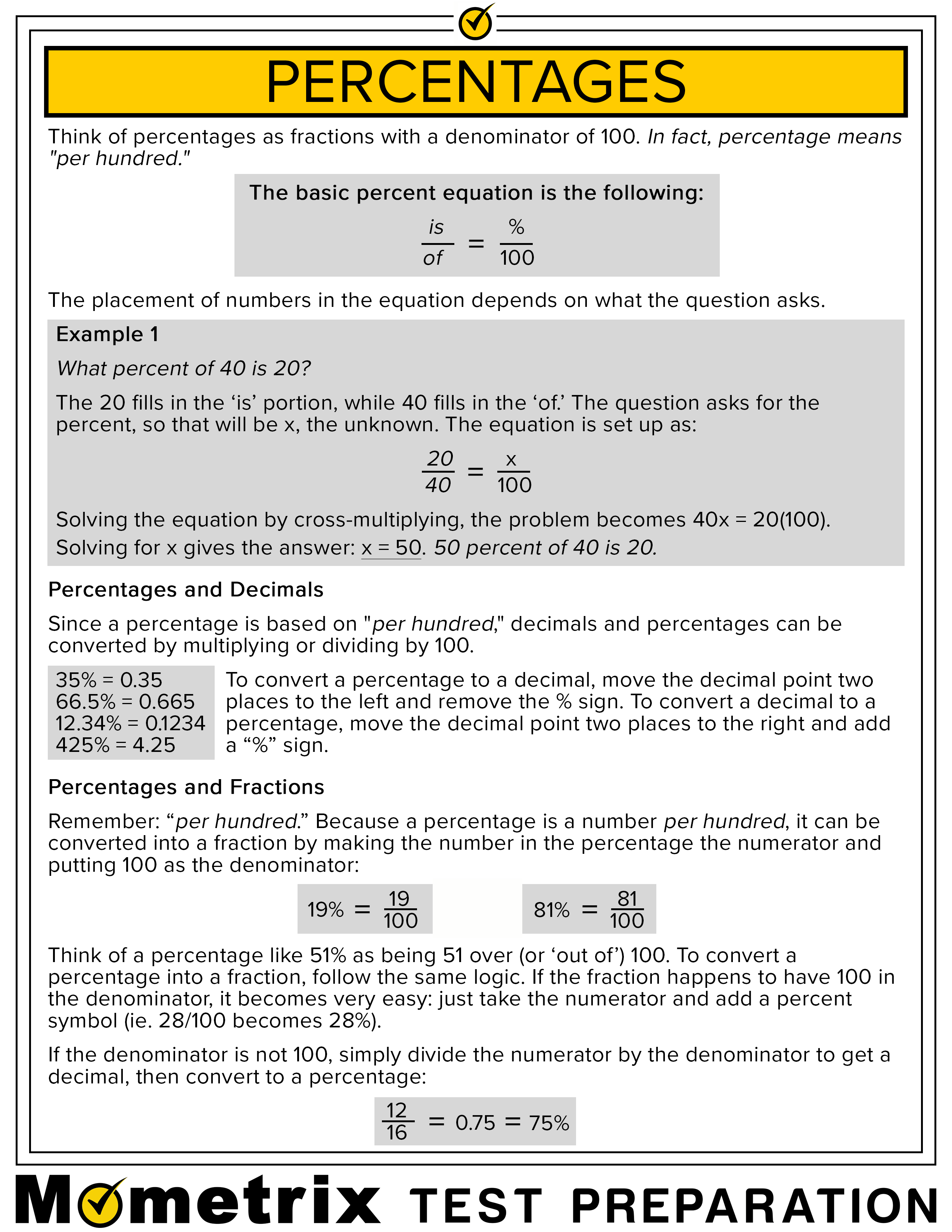# How to Calculate Percentage

## Free Percentages Fact Sheet

Use the percentages fact sheet below to help you get a better understanding of how percentages work. You are encouraged to print or download the percentages fact sheet with the PDF link at the bottom of the page.## Percentages

Hi and welcome to this Mometrix video about percentages. In this video, we’ll discover what a percentage is and how we can calculate and determine a percentage using math.

People use the words percent and percentages interchangeably, even though they have different meanings and are written differently in a sentence.

The Latin term for percent is Per Centum, which means “per 100.” That’s why a percent is a specified number per 100.

The American Heritage dictionary defines a percentage as a “result obtained by multiplying a quantity by a percent.” Let’s take a look at an equation to see what that means.

25 percent of 60 baseballs equals 15 baseballs.

The quantity of baseballs is 60. The percent is 25. Therefore, the percentage is 15. We get this percentage by multiplying the quantity by the percent. (60 x 25%, or 60 x 0.25)

Things become more confusing when people talk about a “percentage point.” A “percentage point” is the portion, or unit, of 1 percent. That means 2.35 would be a percentage point since .35 is the unit of one percent.

Now let’s take a look at percentages in action.

In today’s technology-driven world, it’s easy to get a calculator to do the math for you. But sometimes that actually takes longer than it would to just do it in your head – in some cases. Here, we’ll look at the formula for doing basic math calculations the old fashioned way.

Let’s say you want to calculate 25% of 100. Here’s the equation:

25% = 25100.

Then, 25100x 100 = 25

Remember, you multiply the numerator, the top number, by the 1 in 100. Remember, to get the percentage, you’re multiplying by the hundredth. In this case, that’s the “1” of the “100.” So, 25 multiplied by 1 equals 25.
Let’s do another and calculate 10 percent of 80.

10% = 10100 x 80 = 8

Remember, the denominator — that’s the bottom number — is in hundredths. So, in this case, one 1 multiplied by 8 equals 8.

Now these are very basic equations, but you can see the methods used to calculate the answer.

You can present that answer in different ways, as either a number, fraction, or decimal.

When using a number, you write out the word or use the percent sign. That means it’s either 25 percent of 25%.

When using a decimal, you write 0.25.

And, if you’re writing as a fraction, you write 14. Why one-fourth? Because 25 goes into 100 four times.

So that’s our look percentages and how to use them. Percentages are just like anything else in math. Some are very easy, and some are very complicated.

I hope this overview was helpful!

See you guys next time!

141911

by Mometrix Test Preparation | Last Updated: June 3, 2020